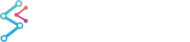﻿ Renko, Volume, Tick and Range Bars | WPF Chart DocumentationSciChart WPF 2D Charts > Stock Charts API > Renko, Volume, Tick and Range Bars
Renko, Volume, Tick and Range Bars

Using the Filters API, you can now create Renko, Volume, Tick and Range bars natively with SciChart WPF. To do this, we use the Aggregation Filters.To see an examples of creating Tick, Range, Volume or Renko Bars, see the Featured Apps > Financial Charts > Aggregation Filters example in the SciChart WPF v5 Examples Suite.

Aggregation Filters Example
Copy Code
```using SciChart.Charting.Model.Filters;
// ...

// 1. Given a List<Tick> where Tick is defined as
public class Tick
{
public Tick()
{
}
public Tick(DateTime date, double open, double high, double low, double close, long volume)
{
DateTime = date;
Open = open;
High = high;
Low = low;
Close = close;
Volume = volume;
}
public DateTime DateTime { get; set; }
public double Open { get; set; }
public double High { get; set; }
public double Low { get; set; }
public double Close { get; set; }
public long Volume { get; set; }
}
OhlcDataSeries<DateTime, double>() _priceData = new OhlcDataSeries<DateTime, double>();
_priceData.Append(_ticks.Select(x=>x.DateTime), _ticks.Select(x => x.Open), _ticks.Select(x => x.High), _ticks.Select(x => x.Low), _ticks.Select(x => x.Close));
// 3. Now aggregate using the FilterExtensions .AggregateBy* extension methods
private void UpdatePriceChart(AggregationPriceChart barStyle)
{
switch (barStyle)
{
case AggregationPriceChart.Count:
PriceSeries = (IOhlcDataSeries<DateTime, double>)_priceData.AggregateByCount(_selectedCount);
break;
case AggregationPriceChart.Time:
PriceSeries = (IOhlcDataSeries<DateTime, double>)_priceData.AggregateByTime(TimeSpan.FromMinutes(_selectedTimeFrame));
break;
case AggregationPriceChart.Volume:
PriceSeries = (IOhlcDataSeries<DateTime, double>)_priceData.AggregateByVolume(_ticks.Select(x => x.Volume.ToDouble()).ToList(), _selectedVolume);
break;
case AggregationPriceChart.Range:
PriceSeries = (IOhlcDataSeries<DateTime, double>)_priceData.AggregateByRange(_selectedRange);
break;
case AggregationPriceChart.Renko:
PriceSeries = (IOhlcDataSeries<DateTime, double>)_priceData.AggregateByRenko(_selectedBrickSize);
break;
}

// Further filters may be applied such as Moving averages using the Filters API
Sma50Series = (IXyDataSeries<DateTime, double>)((IXyDataSeries<DateTime, double>) PriceSeries.ToMovingAverage(50));
Sma50Series.SeriesName = "50 SMA";
}
```